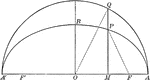### Corresponding Points in an Ellipse and Circle

Illustration of half of an ellipse. "The ordinates of two corresponding points in an ellipse and its…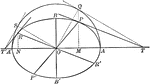### Conjugate Diameters of an Ellipse

Illustration showing that if one diameter is conjugate to a second, the second is conjugate to the first.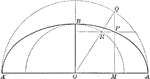### Construction of an Ellipse

Illustration of half of an ellipse and its auxiliary circle used to construct an ellipse by points,…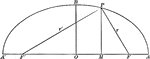### Focal Radii of an Ellipse

Illustration of half of an ellipse. "If d denotes the abscissa of a point of an ellipse, r and r' its…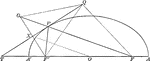### Line Bisecting Angle Between Focal Radii on Ellipse

Illustration of half of an ellipse. "If through a point P of an ellipse a line is drawn bisecting the…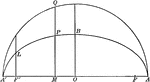### Ordinate and Major Axis of Ellipse

Illustration of half of an ellipse. The square of the ordinate of a point in an ellipse is to the product…### Parallel Tangents to an Ellipse

Illustration showing that tangents drawn at the ends of any diameter are parallel to each other.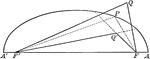### Point Distances to Foci on Ellipse

Illustration of half of an ellipse. "The sum of the distances of any point from the foci of an ellipse…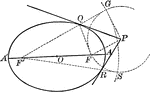### Tangent From External Point to an Ellipse

Illustration of how to draw a tangent to an ellipse from an external point.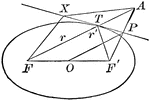### Tangent to an Ellipse

Diagram an ellipse with a tangent line that illustrates "A line through a point on the ellipse and bisecting…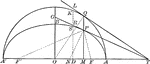### Tangents to an Ellipse

Illustration showing the tangents drawn at two corresponding points of an ellipse and its auxiliary…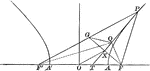### Line Bisecting Angle Between Focal Radii in Hyperbola

Illustration of a hyperbola with a line bisecting the focal radii. "If through a point P of an hyperbola…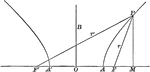### Point on a Hyperbola

Illustration of a point on a hyperbola. "If d denotes the abscissa (x-coordinate) of a point of an hyperbola,…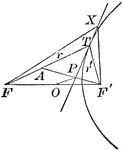### Tangent to a Hyperbola

Diagram part of a hyperbola with a tangent line that illustrates "A line through a point on the hyperbola…